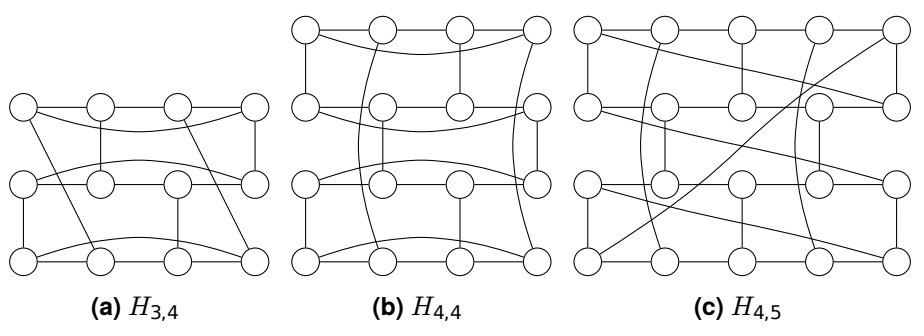시간 제한메모리 제한제출정답맞힌 사람정답 비율
2 초 512 MB0000.000%

## 문제

The Saturn bee (Apis saturnii) is quite an interesting species. To begin with, they build their hives in the shape of a ring. More precisely, a beehive is a hexagonal grid, which we represent as a graph where walls are edges and wall joins are vertices. If we flatten each hexagon a bit so that it becomes a 1 × 2 rectangle, then we can assign an integer coordinate to each vertex so that vertex (i, j) is adjacent to vertices (i, j −1), (i, j +1) and either (i+1, j) if i+j is odd, or (i−1, j) if i + j is even.

To make an n × m grid into a ring the edges wrap around. So, if n and m are even, an edge with endpoint (n, j) will end at (0, j) instead, and an edge with endpoint (i, m) will end at (i, 0). If either coordinate is odd the bees need to twist the grid so that both sides will match: if n is odd then (n, j) becomes (0, j + 1), and if m is odd then (i, m) becomes (i + 1, 0). The swarm mind is aware of the handshaking lemma and does not try to build beehives where both n and m are odd. See Figure A.1 for a few examples of beehives.Figure A.1: Example beehives

Another outstanding fact about Saturn bees is how they guard their hive. Each soldier bee sits on top of a vertex and its task is to control that vertex and the 3 adjacent vertices. The swarm mind is aware that nm/4 bees are required for this, hence this is the number of soldiers in the swarm, but unfortunately some beehives are turning tricky to guard and the Saturn bees refuse to live there.

Your task is to determine whether a beehive is a suitable home for a swarm.

## 입력

The first line of input contains two integers, n and m (2 ≤ m, n ≤ 10 000, m or n even).

## 출력

Output “possible” if nm/4 bees can guard an n × m beehive, and “impossible” otherwise.

## 예제 입력 1

4 6


## 예제 출력 1

impossible


## 예제 입력 2

6 4


## 예제 출력 2

possible


## 출처• 문제를 만든 사람: Marc Vinyals